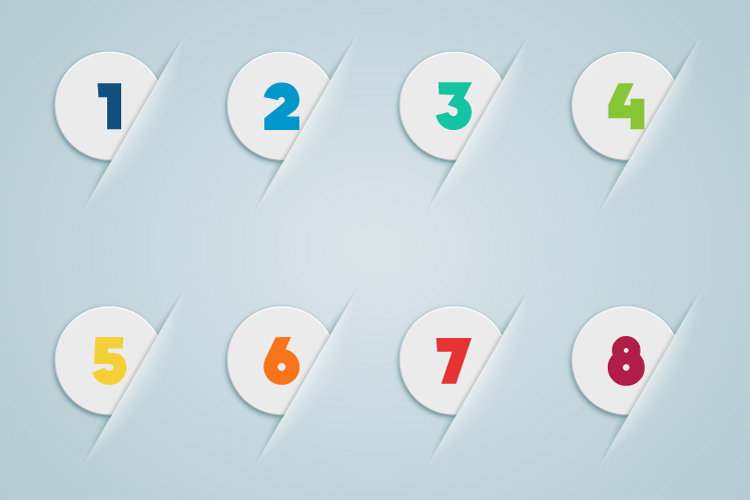# C Program to Count Number of Digits in an Integer

In this example, you will learn to count the number of digits in an integer entered by the user.To understand this example, you should have the knowledge of the following C programming topics:

This program takes an integer from the user and calculates the number of digits. For example: If the user enters 2319, the output of the program will be 4.

## Program to Count the Number of Digits

``````#include <stdio.h>
int main() {
long long n;
int count = 0;
printf("Enter an integer: ");
scanf("%lld", &n);

while (n != 0) {
n /= 10;     // n = n/10
++count;
}

printf("Number of digits: %d", count);
}

``````

Output

```Enter an integer: 3452
Number of digits: 4
```

The integer entered by the user is stored in variable n. Then the `while` loop is iterated until the test expression `n! = 0` is evaluated to 0 (false).

• After the first iteration, the value of n will be 345 and the `count` is incremented to 1.
• After the second iteration, the value of n will be 34 and the `count` is incremented to 2.
• After the third iteration, the value of n will be 3 and the `count` is incremented to 3.
• After the fourth iteration, the value of n will be 0 and the `count` is incremented to 4.
• Then the test expression of the loop is evaluated to false and the loop terminates.